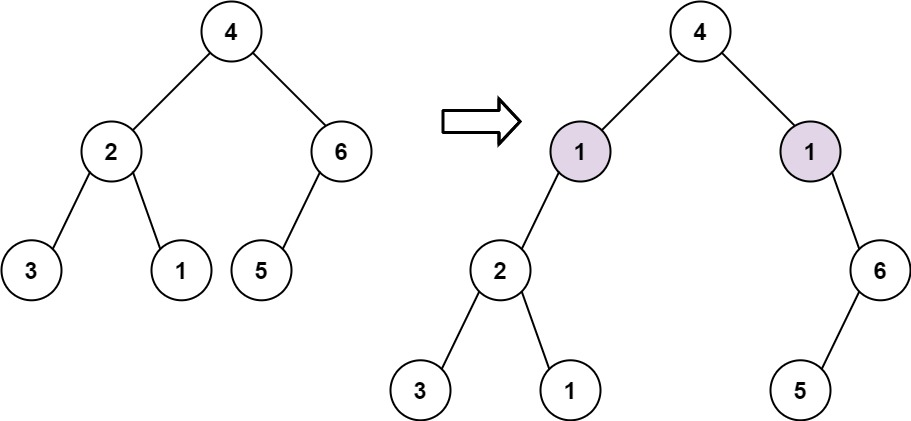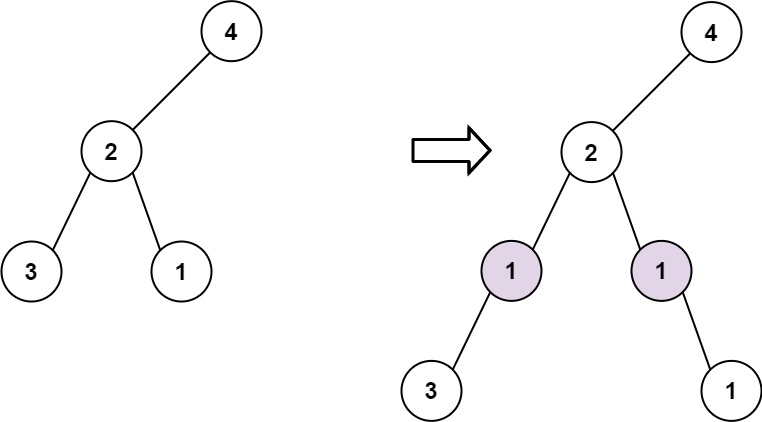### LeetCode• ㊗️
• 大家
• offer
• 多多！

## Problem

Given the root of a binary tree and two integers val and depth, add a row of nodes with value val at the given depth depth.

Note that the root node is at depth 1.

• Given the integer depth, for each not null tree node cur at the depth depth - 1, create two tree nodes with value val as cur’s left subtree root and right subtree root.
• cur’s original left subtree should be the left subtree of the new left subtree root.
• cur’s original right subtree should be the right subtree of the new right subtree root.
• If depth == 1 that means there is no depth depth - 1 at all, then create a tree node with value val as the new root of the whole original tree, and the original tree is the new root’s left subtree.

Example 1:Input: root = [4,2,6,3,1,5], val = 1, depth = 2
Output: [4,1,1,2,null,null,6,3,1,5]


Example 2:Input: root = [4,2,null,3,1], val = 1, depth = 3
Output: [4,2,null,1,1,3,null,null,1]


Constraints:

• The number of nodes in the tree is in the range $[1, 10^4]$.
• The depth of the tree is in the range $[1, 10^4]$.
• -100 <= Node.val <= 100
• $-10^5 <= val <= 10^5$
• 1 <= depth <= the depth of tree + 1

## Code

class Solution {
public TreeNode addOneRow(TreeNode root, int val, int depth) {
if(depth == 1) {
TreeNode newRoot = new TreeNode(val);
newRoot.left = root;
return newRoot;
}

queue.offer(root);

int currDepth = 1;
while(!queue.isEmpty()) {
int size = queue.size();
for(int i = 0; i < size; i++) {
TreeNode curr = queue.poll();
TreeNode left = curr.left;
TreeNode right = curr.right;

if(currDepth == depth - 1) {
curr.left = new TreeNode(val);
curr.left.left = left;

curr.right = new TreeNode(val);
curr.right.right = right;
} else {
if(left != null) queue.offer(left);
if(right != null) queue.offer(right);
}
}

currDepth++;
}

return root;
}
}

class Solution {
public TreeNode addOneRow(TreeNode root, int val, int depth) {
if(depth <= 1) {
TreeNode newRoot = new TreeNode(val);
if(depth == 1) newRoot.left = root;
// -1 is a flag to attach right node
if(depth == -1) newRoot.right = root;
return newRoot;
}

if(root == null) return null;

root.left  = addOneRow(root.left,  val, depth - 1);
if(depth == 2) {